Question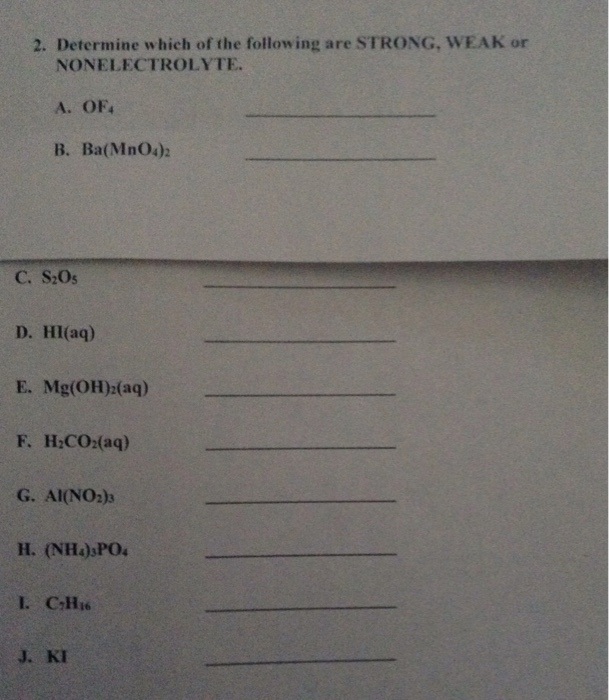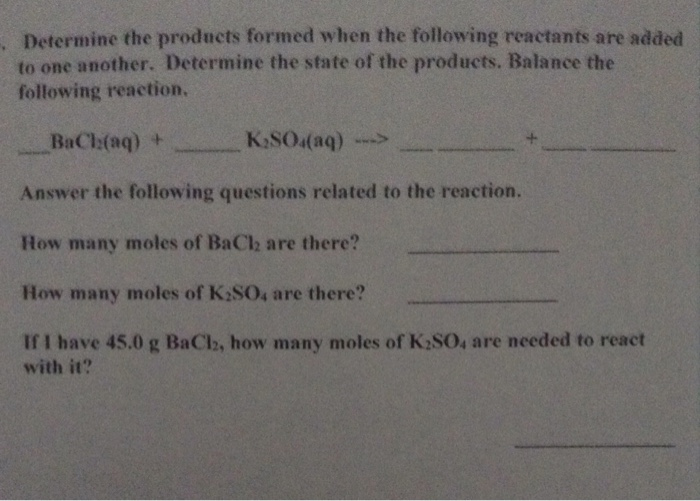1) OF4--------doe snot exist

2) Ba(MnO4)2----------------May be non eletcrolyte or week electrolyte(Since the eletronegative difference is considerably less between Ba and Mn)

3) S2O5---------------------Non electrolyte for the same reason mentioned above

4) HI------------------Strong electrolyte (Ionic compound)

5) Mg(OH)2aq------------------------Strong electrolyte (Ionic compound)

6) H2C2O2 (aq) ------------------------------- weel electrolyte

7) Al(NO2)3---------------------------Strong electrolyte

8) (NH4 )3(PO4)--------------------------- Week electrolyte

9) C7H16------------------------non electrolyte

10) KI----------------------Strong elctrolyte#### Earn Coins

Coins can be redeemed for fabulous gifts.

Similar Homework Help Questions
• ### 2. What type of compounds (in general) are a. Nonelectrolyte b. Weak electrolyte c. Strong electrolyte...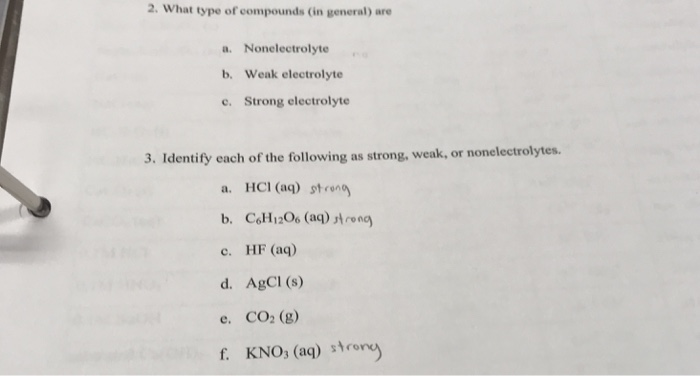2. What type of compounds (in general) are a. Nonelectrolyte b. Weak electrolyte c. Strong electrolyte 3. Identify each of the following as strong, weak, or nonelectrolytes. a. HCl(aq) strong b. CH1206 (aq) strong c. HF (aq) d. AgCl(s) e. CO2 (g) f. KNO3 (aq) strony

• ### aent 6: L B. Testing the conductivity of reactions a OIM KNO, and O. IM Na,CO Species Present Strong, weak or nonel...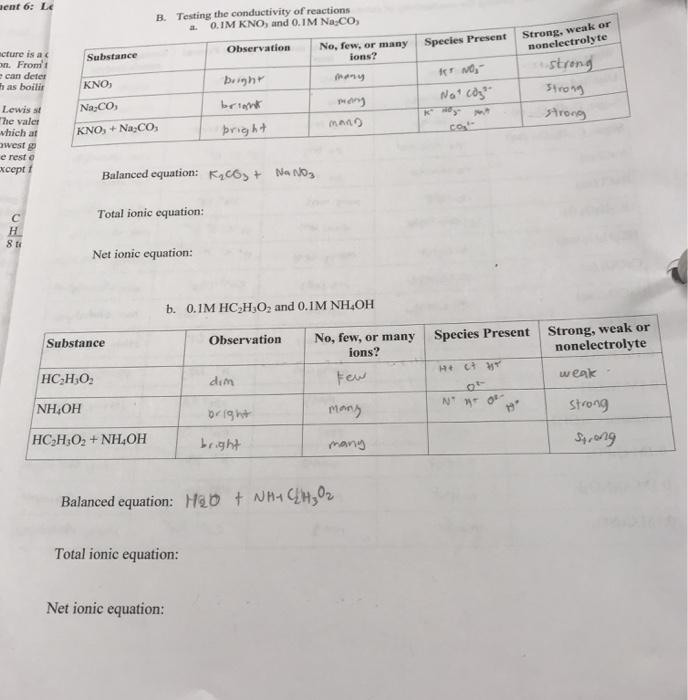aent 6: L B. Testing the conductivity of reactions a OIM KNO, and O. IM Na,CO Species Present Strong, weak or nonelectrolyte Observation No, few, or many lons? Substance ncture is ac on. From can deter has boilir strong hany bright KNO It No Narcos Strong Na CO briont strong MA KNO, +Na:CO; pright Lewis st The valer which at west e resto xcept f Balanced equation: K2CO3 + NA NO Total ionic equation: Net ionic equation: b. 0.1M HC2H2O2...

• ### Classify HF as a strong or weak acid or base in aqueous solution. Weak base O...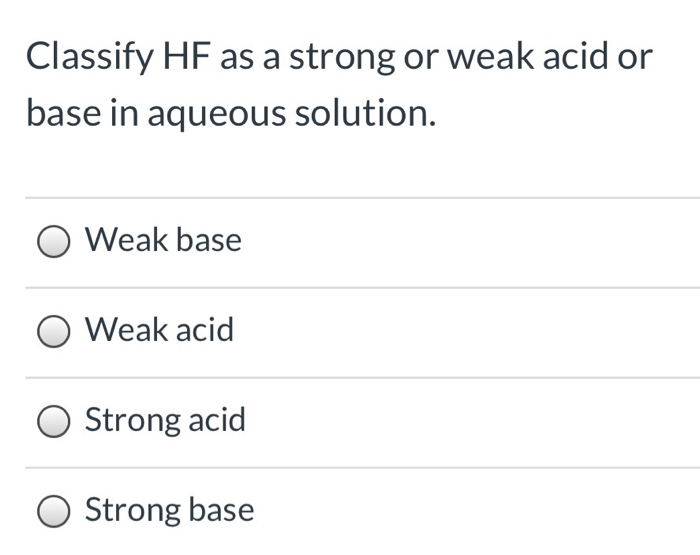Classify HF as a strong or weak acid or base in aqueous solution. Weak base O Weak acid O Strong acid Strong base Which of the following is the correct molecular equation for the following reaction? HC2H302 (aq) Ba(OH)2 (aq) O 2 H (aq) + Ba(ОН)2 (s) +2 H20 ) +2 C2H302 (aq) +2 C2H302 Ba2+ (aq) (aq) Ba2+ (aq) 2 OH O 2 H(aq)+2 C2H302 (aq) Ba2 (aq) +2 C2H3O2 (aq)+ 2 H20 (aq) (1) +Ba(OH)2 (aq) O 2...

• ### 1. The following pictures represent solution at various points in the titration of a weak acid...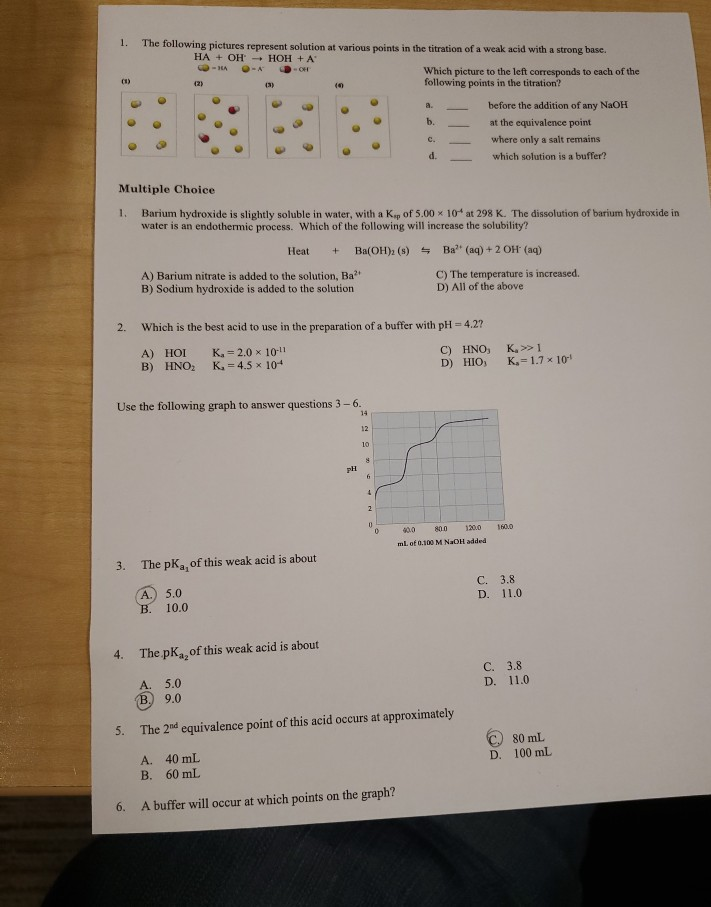1. The following pictures represent solution at various points in the titration of a weak acid with a strong base. HA + OH - HOH + A OH - -OH Which picture to the left corresponds to cach of the following points in the titration? before the addition of any NaOH at the equivalence point where only a salt remains which solution is a buffer? Multiple Choice 1. Barium hydroxide is slightly soluble in water, with a Kap of 5.00...

• ### pls do all Page 6 of 14 22) Identify a good buffer. ) small amounts of both a weak acid and its conjugate base B) significant amounts of both a strong acid and a strong bso C) small amounts of bot...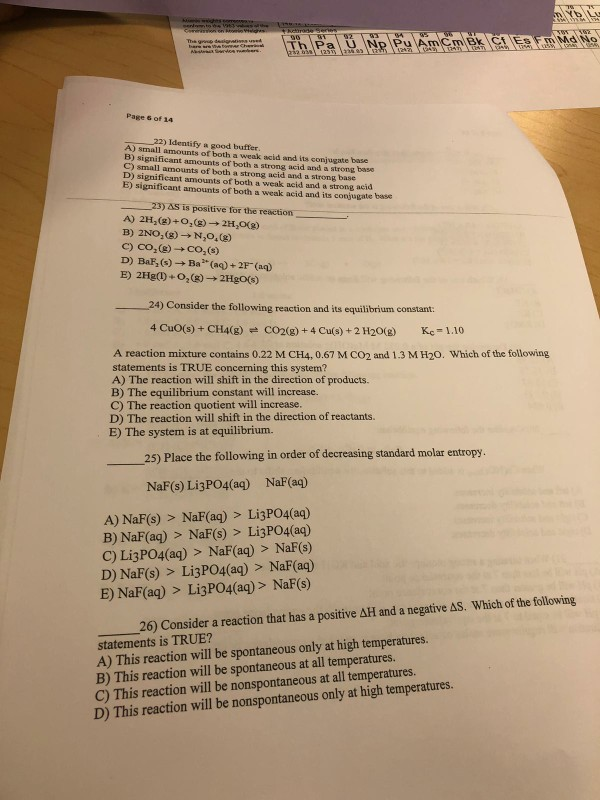pls do all Page 6 of 14 22) Identify a good buffer. ) small amounts of both a weak acid and its conjugate base B) significant amounts of both a strong acid and a strong bso C) small amounts of both a strong acid and a strong base D) significant amounts of both a weak acid and a strong acid E) significant amounts of both a weak acid and its conjugate base 23) AS is positive for the reaction A)...

• ### m water, one of the following electrolyte, exists only as ions (100%) in solution a) nonconductor...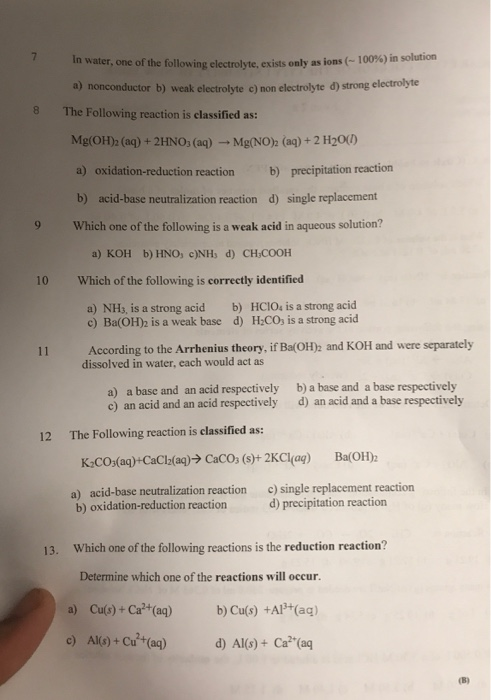m water, one of the following electrolyte, exists only as ions (100%) in solution a) nonconductor b) weak electrolyte c) non electrolyte d) strong electrolyte The Following reaction is classified as: Mg(OH)2 (aq) + 2HNO3(aq) - Mg(NO)2 (aq) + 2 H20(1 a) oxidation-reduction reaction b) precipitation reaction b) acid-base neutralization reaction d) single replacement 9 Which one of the following is a weak acid in aqueous solution? a) KOH b) HNO3 c)NH; d) CH-COOH 10 Which of the following is...

• ### 1. Identify the following compounds as a strong or weak electrolyte. a) HF b) AgPO4 c)...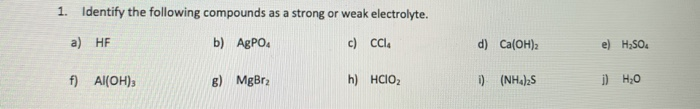1. Identify the following compounds as a strong or weak electrolyte. a) HF b) AgPO4 c) Cle d) Ca(OH)2 e) H2SO4 f) Al(OH) 6) MgBr? h) HCIO \) (NH4)2S 1) но 2. Write the complete ionic equation and the net ionic equation for the neutralization reaction between sulfuric acid and potassium hydroxide. Molecular equation: Complete ionic equation: Net ionic equation: I

• ### 11) Determine the form ula weight of the following a) HSO b) Ha c) NaoH d)...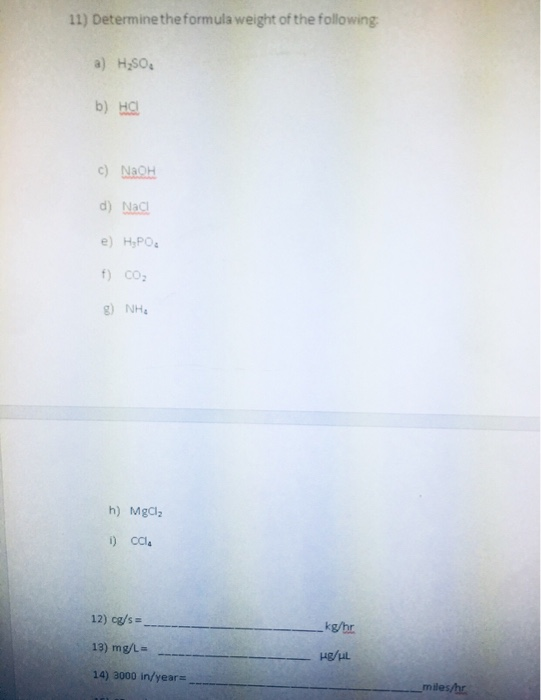11) Determine the form ula weight of the following a) HSO b) Ha c) NaoH d) Nac e) HPO f) cO g) NH h) MgCl i) CCl 12) cg/s kg/hr 13) mg/L 14) 3000 in/year miles/hr

• ### help with thes 12. In the reaction, K2SO4(99) + Ba(NO3)2(aq) + BaSO4(s) + 2 KNO3(aq), which...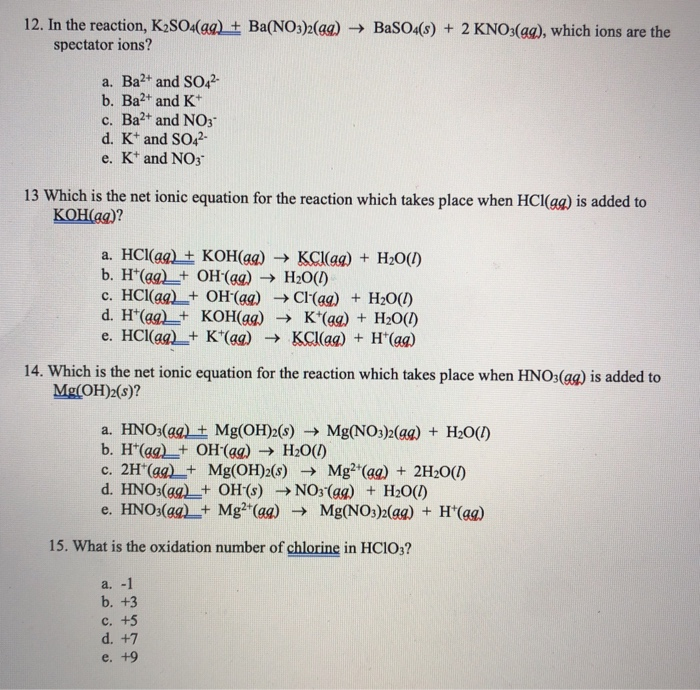help with thes 12. In the reaction, K2SO4(99) + Ba(NO3)2(aq) + BaSO4(s) + 2 KNO3(aq), which ions are the spectator ions? a. Ba2+ and SO42- b. Ba2+ and K+ c. Ba2+ and NO3 d. K+ and SO42- e. K+ and NO3 13 Which is the net ionic equation for the reaction which takes place when HCl(ag) is added to KOH(aq)? a. HCl(aq) + KOH(99) KCl(aq) + H2O(1) b. H(aq) + OH(aq) + H2O(1) c. HCl(aq) + OH(aq) + Cl(aq) +...

• ### please write legible 1. Identify each of the following reactions as a precipitation reaction, an acid-base...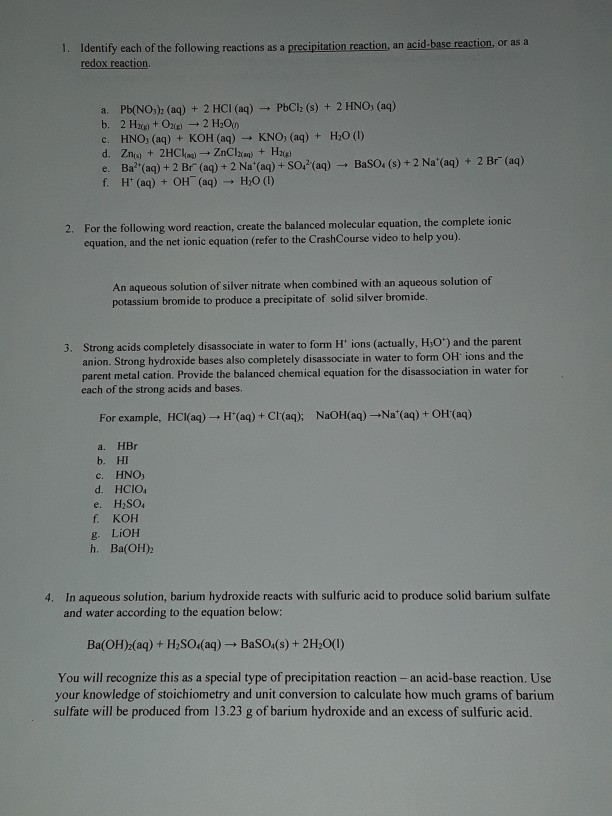please write legible 1. Identify each of the following reactions as a precipitation reaction, an acid-base reaction, or as a redox reaction + a. Pb(NO3)2 (aq) + 2 HCl(aq) PbCl (s) + 2 HNO, (aq) b. 2 Hxe + O2 + 2 H2O c. HNO, (aq) + KOH(aq) KNO, (aq) + H20 (1) d. Zna + 2HCl(a) - ZnClaraq) + Halle e. Ba?'(aq) + 2 Br(aq) + 2 Na(aq) + SO/"(aq) BaSO. (s) + 2 Na'(aq) + 2 Br" (aq)...